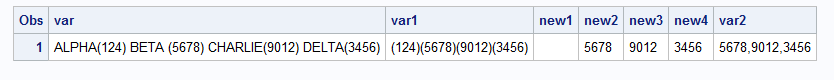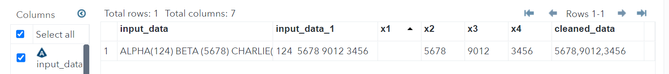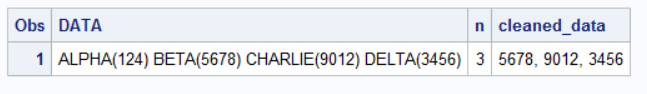## How to extract multiple 4-digit numbers from a string and separate them by using a Comma delimiter?

Hi folks, I ran into a situation where I need to extract 4-digit numbers from a string. The location of these numbers varies and sometimes there would also be 3-digit or 5-digit numbers along with (sometimes without) 4-digit number. Once extracted, these number needs to be separated by a delimiter like comma (,).

Example: - Original data

 DATA ALPHA(124) BETA (5678) CHARLIE(9012) DELTA(3456)

Data after extracting numbers and separated by delimiter like comma.

 DATA CLEANED_DATA ALPHA(124) BETA(5678) CHARLIE(9012) DELTA(3456) 5678, 9012, 3456

As you see in the above example the 4-digit numbers in DATA string are extracted to CLEANED_DATA variable and are separated by comma delimiter. It would be great if you could help me with this.

Banana19

7 REPLIES 7

## Re: How to extract multiple 4-digit numbers from a string and separate them by using a Comma delimit

So your example implies that 3-digit values are not to be included, correct? and the same for 5-digit?

Critical: is the value always inside parentheses? If not this going be a bit more challenging.

Do you have any  clue at all what the maximum number of 4-digit values are involved? You will need to specify a length of the variable to receive them.

Style comment: I have a very hard time considering any variable that holds two or more values as "clean". To use that "cleaned_data" you still have to parse out each value for any real purpose.

One approach:

```data example;
var="ALPHA(124) BETA(5678) CHARLIE(9012) DELTA(3456)";
length word \$ 25 cleaned_data \$ 100;
do i=1 to countw(var,'()');
word=scan(var,i,'()');
if length(word)= 4 and notdigit(strip(word))=0 then cleaned_data=catx(',',cleaned_data,word);
end;
run; ```

This assumes that the value is always inside ().

"guess" at lengths for the "words" in the value and the overall result variable.

Countw function returns the number of "words" in the first parameter position. The second parameter represents characters to use as delimiters between words.

Scan extracts a single "word". The first parameter is the value to search, the second the number of the "word" desired and third is the delimiters between words.

Length returns the length of extracted word and in any of the characters in the Striped value of the word (removes any leading or trailing blanks) are not digits then the result other than 0 indicates something not a digit was found.

Catx function places the first parameter, your comma, between values .

The Countw and Scan are not looking at PAIRS of (), but each occurrence of any of the characters. So you have poor tying and get values like ((1234) it should work. But again, this approach does require that all the values always are inside quotes with no other characters except spaces.

## Re: How to extract multiple 4-digit numbers from a string and separate them by using a Comma delimit

The simplest way, using data step character functions.
e.g.

``````data have;
var= 'ALPHA(124) BETA (5678) CHARLIE(9012) DELTA(3456)';
var1= compress(var, '', 'a');
call symputx('num', countc(var, ')'));
proc print;run;

data want;
set have;
array new [*] \$ new1-new&num;
do i=1 to &num;
new{i}= compress(scan(var1, i, ')'), '', 'p');
if length(new{i}) le 3 then new{i}='';
end;
var2= catx(',', of new:);    drop i;    ````proc print;run;````## Re: How to extract multiple 4-digit numbers from a string and separate them by using a Comma delimit

data new;
input_data = 'ALPHA(124) BETA (5678) CHARLIE(9012) DELTA(3456)';
input_data_1 = trim(left(compress(input_data, '', 'kd')));  /* kd keeps only digits */

array x \$20.;

do i = 1 to 4;
x[i] = scan(input_data_1, i, '');

if length(x{i}) ne 4 then x{i}='';
end;
cleaned_data= catx(',', of x:);
drop i;

run;Ksharp
Super User

## Re: How to extract multiple 4-digit numbers from a string and separate them by using a Comma delimit

``````data example;
var="ALPHA(124) BETA(5678) CHARLIE(9012) DELTA(3456)";
run;

data temp;
set example;
n+1;
pid=prxparse('/\(\d\d\d\d\)/');
s=1;e=length(var);
call prxnext(pid,s,e,var,p,l);
do while(p>0);
temp=substr(var,p,l);output;
call prxnext(pid,s,e,var,p,l);
end;
keep n var temp;
run;
data want;
do until(last.n);
set temp;
by n;
length want \$ 200;
want=catx(',',want,compress(temp,'()'));
end;
drop temp;
run;``````

## Re: How to extract multiple 4-digit numbers from a string and separate them by using a Comma delimit

@Ksharp has the right idea here.

Let's explain what this code is doing and tweak it to generated the desired results directly.

So it is using the CALL PRXNEXT() function to repeatedly call the same Regular Expression and return a POSITION  pointer to where the next matched substring starts in the string.

Here is the syntax description from the SAS documentation.

CALL PRXNEXT(regular-expression-idstartstopsourcepositionlength);

Here is the regular expression being used

``/\(\d\d\d\d\)/``

Which means to look for a 6 character string that has 4 digits with parentheses on the outside.

So let's rewrite the code to use variable names that look like the names used in the syntax example from the documentation so it is a little clearer what is what.

And let's add some code to pull out just the digits (without the parentheses) by changing the SUBSTR() function call and concatenate them into the desired string by using the CATX(). Since we know the matched substring has 4 digits you can just use SUBSTR(DATA,POSITION+1,4) to skip the ( and read just the 4 digits.

``````data example;
input DATA \$80.;
cards4;
ALPHA(124) BETA(5678) CHARLIE(9012) DELTA(3456)
;;;;

data want;
set example;
length n 8 cleaned_data \$80 ;
if _n_=1 then regex_id=prxparse('/\(\d\d\d\d\)/');
retain regex_id ;
start=1;
end=length(DATA);
call prxnext(regex_id,start,end,DATA,position,length);
do n=0 by 1 while(position>0);
cleaned_data=catx(', ',cleaned_data,substr(DATA,position+1,4));
call prxnext(regex_id,start,end,DATA,position,length);
end;
drop regex_id start end position length ;
run;``````

Results:## Re: How to extract multiple 4-digit numbers from a string and separate them by using a Comma delimit

Hi @Tom ,

It's nice of you to give an explanations to every single statement. Like me, most of the Community members must have appreciated your time and effort to help others.

As  a new starter in Regular Expressions, I have  a question on your comment above:

Here is the regular expression being used

``/\(\d\d\d\d\)/``

Which means to look for a 6 character string that has 4 digits with parentheses on the outside.

What I see here (according to manuals) is:
/.../  - start and end of regular expression
(...) - grouping of values
\d\d\d\d - 4 digits
\ (the very first forward slash outside the parenthesis) - Overrides the next metacharacter such as a ( or ?

I'm wondering which metacharacter or which part of reg.expression here specifies 6 character string ?
Or would it be incorrect to write the statement as /(\d\d\d\d)/   or  /(\d{4})/    ?
Trying to understand the expressions advantages one over another.
Thank you!

## Re: How to extract multiple 4-digit numbers from a string and separate them by using a Comma delimit

You have your statements out of order and that is the source of your confusion.

/.../  - start and end of regular expression
\ (  - Match a (.  The backwards slash means the ( is NOT a metacharacter.
\d\d\d\d - Match 4 digits
\) - Match a ).  The backwards slash means the ) is NOT a metacharacter.

Discussion stats
• 7 replies
• 740 views
• 17 likes
• 6 in conversation